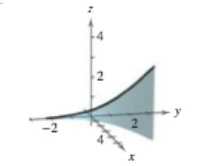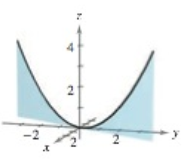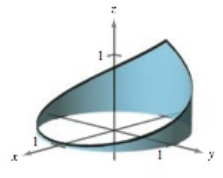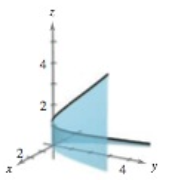Chapter 12.1, Problem 20E

Chapter
Section
Textbook Problem

# MatchingIn Exercises 19–22, match the equation with its graph. [The graphs are labeled (a), (b), (c), and (d).](a)(b)(c)(d)r ( t ) = cos ( π t ) i + sin ( π t ) j + t 2 k ,       − 1 ≤ t ≤ 1

To determine
Match the graph of the function, r(t)=cos(πt)i+sin(πt) j+t2 k from the provided four options.

Explanation

Given:

The equation r(t)=cos(πt)i+sin(πt) j+t2 k where 1t1. The graph the curve are shown below,

Explanation:

Consider the provided vector equation,

r(t)=cos(πt)i+sin(πt) j+t2 k where 1t1

Draw the space curve of the provided vector equation by the use of maple software:

Step1: First write the library function with(VectorCalculus):.

Step2: Define the position vector in Cartesian coordinate system,

S1:=PositionVecor([cos(πt),sin(πt),t2],cartesian)

Step3: Press Enter button

### Still sussing out bartleby?

Check out a sample textbook solution.

See a sample solution

#### The Solution to Your Study Problems

Bartleby provides explanations to thousands of textbook problems written by our experts, many with advanced degrees!

Get Started

#### Convert the expressions in Exercises 8596 radical form. 45x3/2

Finite Mathematics and Applied Calculus (MindTap Course List)

#### Add and reduce to lowest terms. 4514+1659+13

Contemporary Mathematics for Business & Consumers

#### Find the angle between a cubes diagonal and one of its edges.

Calculus: Early Transcendental Functions (MindTap Course List)

#### For

Study Guide for Stewart's Multivariable Calculus, 8th

#### True or False: A straight (simple) substitution may be used to evaluate 2+lnxxdx.

Study Guide for Stewart's Single Variable Calculus: Early Transcendentals, 8th